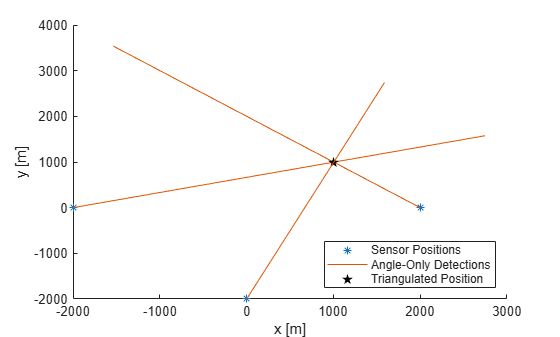Documentation

# triangulateLOS

Triangulate multiple line-of-sight detections

## Syntax

``estPos = triangulateLOS(detections)``
``[estPos,estCov] = triangulateLOS(detections)``

## Description

example

````estPos = triangulateLOS(detections)` estimates the position of a target in a global Cartesian coordinate frame by triangulating a set of angle-only `detections`. Angle-only detections are also known as line-of-sight (LOS) detections. For more details, see Algorithms.```
````[estPos,estCov] = triangulateLOS(detections)` also returns `estCov`, the covariance of the error in target position. The function uses a Taylor-series approximation to estimate the error covariance.```

## Examples

collapse all

Load a MAT-file containing a set of line-of-sight detections stored in the variable `detectionSet`.

`load angleOnlyDetectionFusion.mat`

Plot the angle-only detections and the sensor positions. Specify a range of 5 km for plotting the direction vector. To specify the position of the origin, use the second measurement parameter because the sensor is located at the center of the platform. Convert the azimuth and elevation readings to Cartesian coordinates.

```rPlot = 5000; for i = 1:numel(detectionSet) originPos = detectionSet{i}.MeasurementParameters(2).OriginPosition; az = detectionSet{i}.Measurement(1); el = detectionSet{i}.Measurement(2); [xt,yt,zt] = sph2cart(deg2rad(az),deg2rad(el),rPlot); positionData(:,i) = originPos; plotData(:,3*i+(-2:0)) = [xt yt zt]'.*[1 0 NaN]+originPos; end plot3(positionData(1,:),positionData(2,:),positionData(3,:),'*') hold on plot3(plotData(1,:),plotData(2,:),plotData(3,:))```

Triangulate the detections by using `triangulateLOS`. Plot the triangulated position.

```[estPos,estCov] = triangulateLOS(detectionSet); plot3(estPos(1),estPos(2),estPos(3),'pk','MarkerFaceColor','k') hold off legend('Sensor Positions','Angle-Only Detections','Triangulated Position', ... 'location','southeast') xlabel('x [m]') ylabel('y [m]') view(2)```## Input Arguments

collapse all

Line-of-sight measurements, specified as a cell array of `objectDetection` objects. Each object has the properties listed in the table.

PropertyDefinition
`Time`Measurement time
`Measurement`Object measurements
`MeasurementNoise`Measurement noise covariance matrix
`SensorIndex`Unique ID of the sensor
`ObjectClassID`Object classification
`MeasurementParameters`Parameters used by initialization functions of nonlinear Kalman tracking filters
`ObjectAttributes`Additional information passed to tracker

Each detection must specify the `MeasurementParameters` property as a structure with the fields described in the table.

ParameterDefinition
`Frame`

Frame used to report measurements. Specify `Frame` as `'spherical'` for the first structure.

`OriginPosition`

Position offset of the origin of the frame relative to the parent frame, represented as a 3-by-1 real vector.

`OriginVelocity`

Velocity offset of the origin of the frame relative to the parent frame, represented as a 3-by-1 real vector.

`Orientation`

A 3-by-3 real-valued orthonormal frame orientation matrix.

`IsParentToChild`

A logical scalar that indicates if `Orientation` is given as a frame rotation from the parent coordinate frame to the child coordinate frame. If `false`, then `Orientation` is given as a frame rotation from the child coordinate frame to the parent coordinate frame.

`HasElevation`

A logical scalar that indicates if elevation is included in the measurements. This parameter is `true` by default.

`HasAzimuth`A logical scalar that indicates if azimuth is included in the measurements. This parameter is `true` by default. If specified as a field, it must be set to `true`.
`HasRange`A logical scalar that indicates if range is included in the measurements. This parameter must be specified as a field and set to `false`.
`HasVelocity`

A logical scalar that indicates if velocity is included in the measurements. This parameter is `false` by default. If specified as a field, it must be set to `false`.

The function provides default values for fields left unspecified.

## Output Arguments

collapse all

Estimated position of the target, returned as a 3-by-1 vector.

Estimated error covariance of the target position, returned as a 3-by-3 matrix.

## Algorithms

Multiple angle-only or line-of-sight measurements result in lines in space. These lines might or might not intersect because of measurement noise. `triangulateLOS` uses a suboptimal linear least-squares method to minimize the distance of miss between multiple detections. The formulation makes these assumptions:

• All detections report measurements with approximately the same accuracy in azimuth and elevation (if measured).

• The distances from the different sensors to the triangulated target are all of the same order.